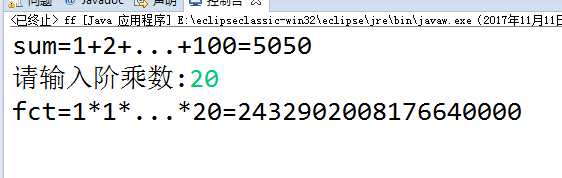# JAVA：计算1+2+...+100的和以及m!

import java.util.Scanner;
public class ff {
public static void main(String[] args) {
// TODO 自动生成的方法存根
int sum=0,n=1,m=0; //进入while循环之前的变量必须初始化
long fct=1; //阶乘的成绩数据可能很大，所以声明为long型
do{
sum +=n;
n++;
}while(n<=100);
System.out.println(“sum=1+2+…+100=”+sum);
Scanner sc=new Scanner(System.in);
System.out.print(“请输入阶乘数:”);
m=sc.nextInt();
n=1; //注意此处给n重新赋值为1的重要性
do{
fct *=n;
n++;
}while(n<=m);
System.out.println(“fct=1*1*…*”+m+”=”+fct);

}


}do…while语句
1）定义：先执行循环体的语句序列，再检查条件，如果条件成立，则再次执行循环体的代码。
2）格式：do {

}
while（<条件表达式>）；